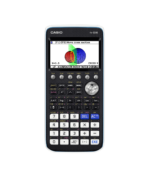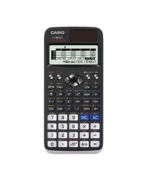Calculus Archives - Casio Calculators

# Calculus

Displaying Resources

FilterHow to Video
fx-CG50 IB A-Level
Quick Skill: Calculations Using Function Notation

How to use function notation to perform operations in the Run-Matrix app. You wi...How to Video
fx-CG50 A-Level IB
Calculus in Mechanics – Derivatives

How to analyse the position equation of a particle to find average, instant and ...How to Video
fx-CG50 A-Level IB
Calculus in Mechanics – Derivative Functions and Integration

How to plot and analyse the relationship between the derivative functions of the...How to Video
fx-CG50 A-Level
Tangents and Normals – Parametric Functions

How to sketch the tangent and normal to a curve in parametric form. Includes fin...How to Video
fx-CG50 IB A-Level
Tangents and Normals – Cartesian Functions

How to sketch the tangent and normal to a curve in Cartesian form. Includes find...How to Video
fx-CG50 A-Level GCSE IB
Table – Calculating Values

How to calculate a table of values for a function. Change the step interval and ...How to Video
fx-CG50 A-Level IB
Integration – Numerical and Graphical

How to numerically evaluate definite integrals of a function. Plot cartesian fun...How to Video
fx-CG50 A-Level IB
Integration – Mixed

How to integrate graphically and numerically between two functions using interse...How to Video
fx-CG50 A-Level IB
Derivatives – Evaluation and Graphing

How to numerically evaluate first and second derivatives of a cartesian function...How to Video
A-Level
Calculus

How to integrate a function between limits to find the definite integral. Differ...How to Leaflet
fx-CG50 A-Level
Integration – Numerical and Graphical (Worksheet)

Worksheet to accompany the video on how to numerically evaluate definite integra...How to Leaflet
fx-CG50 A-Level
Derivatives – Evaluation and Graphing (Worksheet)

Worksheet to accompany the video on how to numerically evaluate first and second...How to Leaflet
fx-991EX A-Level GCSE
Calculate – Maths Quick Start

How to enter basic calculations on the fx-991EX. Includes logarithms summation, ...